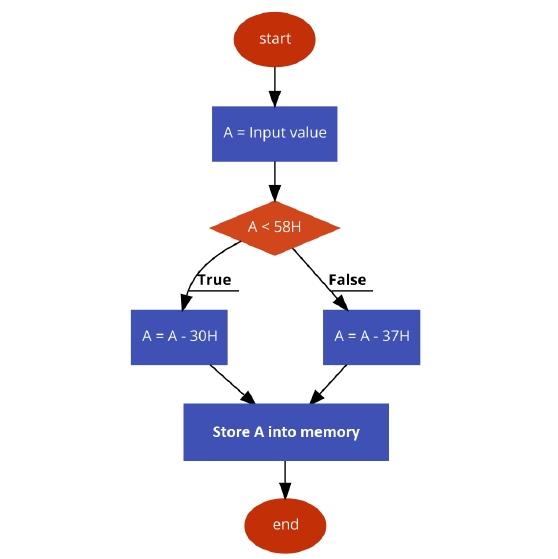# 8085 Program to convert ASCII to binary

Now let us see a program of Intel 8085 Microprocessor. This program will convert ASCII to binary values.

### Problem Statement

Write 8085 Assembly language program to convert ASCII to binary or Hexadecimal character values.

### Discussion

We know that the ASCII of number 00H is 30H (48D), and ASCII of 09H is39H (57D). So all other numbers are in the range 30H to 39H. The ASCII value of 0AH is 41H (65D) and ASCII of 0FH is 46H (70D), so all other alphabets (B, C, D, E, F) are in the range 41H to 46H.

Here the logic is simple. We are checking whether the ASCII value is less than 58H (ASCII of 9+ 1) When the number is less 58, then it is numeric value. So we simply subtract 30H from the ASCII value, and when it is greater than58H, then it is alphabetical value. So for that we are subtracting37H.

first input

Data
.
.
.
.
.
.
8000
41
.
.
.
.
.
.

second input

Data
.
.
.
.
.
.
8000
35
.
.
.
.
.
.

third input

Data
.
.
.
.
.
.
8000
46
.
.
.
.
.
.

### Flow Diagram### Program

HEX Codes
Labels
Mnemonics
F000
21, 00, 80

LXI H, 8000H
F003
7E

MOV A, M
Load ASCII data to Acc from memory
F004
FE, 58

CPI 58H
Compare withASCII(9) + 1
F006
D2, 0E, F0

JNC NUM
The input is numeric
F009
D6, 37

SUI 37H
Subtract offset to get Alphabetic character
F00B
C3, 10, F0

JMP STORE
Store the result
F00E
D6, 30
NUM
SUI 30H
Subtract 30to get numeric value
F010
23
STORE
INX H
Point to next location
F011
77

MOV M,A
Store Acc content to memory
F012
76

HLT
Terminate the program

first output

Data
.
.
.
.
.
.
8001
0A
.
.
.
.
.
.

second output

Data
.
.
.
.
.
.
8001
05
.
.
.
.
.
.

third output

Data
.
.
.
.
.
.
8001
0F
.
.
.
.
.
.